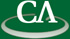## Talk Slides

### Andrew Arnold, School of Computer Science, University of Waterloo

Wednesday September 4th, 2013, 2:30pm, K9509.

```Abstract:

We consider the problem of interpolating a sparse polynomial f, given an
functional representation of f.  Namely, we consider the problem of
reconstructing the terms of f, where f is given by a straight-line program
or arithmetic circuit.  An immediate application of this problem is faster
expansion of some polynomial expressions.  We will discuss recent results
concerning this problem and introduce a new faster Monte Carlo algorithm.

```